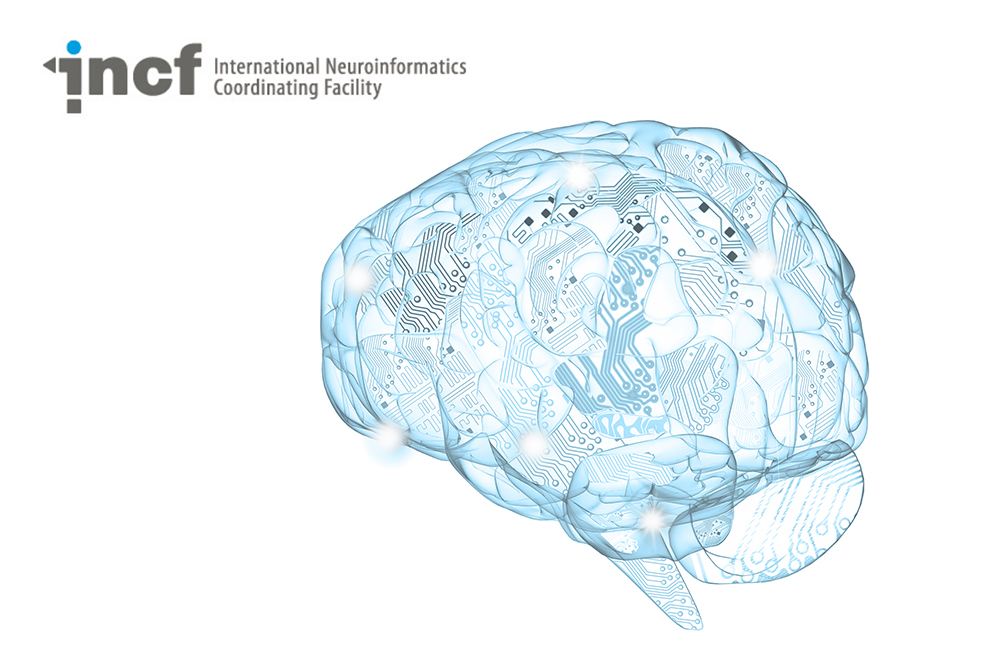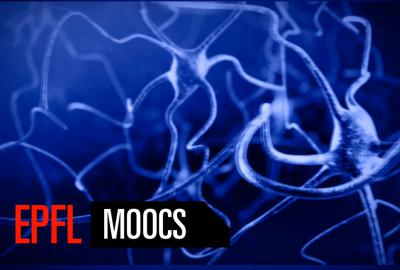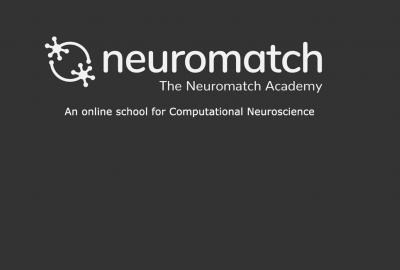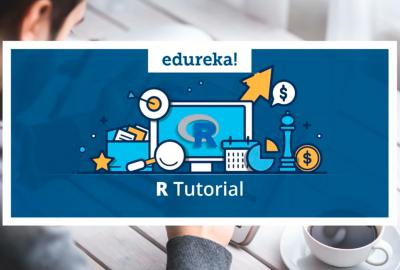# Computational Modeling of Neuronal Plasticity

Category
Level
Intermediate

In this course by Florence I. Kleberg and Prof. Jochen Triesch, you will learn how computational neuroscientists use mathematical models and computer simulations to study different plasticity phenomena in the brain. During the course, you will program your own neuron model, a so-called leaky-integrate-and-re (LIF) neuron model, and simulate it with a computer. Then you will add various neuronal properties and plasticity mechanisms to the model and study how they operate. This course will deepen your understanding of neural plasticity and prepare you for studying plasticity and learning in larger models such as neural networks.

• This course provides users with a brief video introduction to the concepts, lecture notes, and solution figures
• The main idea is to model a neuron and its plasticity mechanisms from scratch, without the use of specialised neuronal modelling software such as Brian, NEURON or NEST. Of course, the use of python packages such as numpy, scipy, ipython and so on is allowed
• It is advised to be familiar with the basics of the Python programming language before commencing the course
Course Features
The Leaky integrate-and-fire neuron
Spike-Timing Dependent Plasticity (STDP)
Homeostasis
Short-term plasticity (STP)
Lessons of this Course
1
1
Duration:
0:40

This lecture 1/15 is part of the Computational Modeling of Neuronal Plasticity Course that aims to teach users how to build a mathematical model of a neuron, its inputs, and its neuronal plasticity mechanisms, by writing your own Python program. This lecture provides users with a brief video introduction to the concepts that serves as a companion to the lecture notes and solution figures.

Authors: Florence I. Kleberg and Prof. Jochen Triesch.

2
2
Duration:
1:23

This lecture (2/15) is part of the Computational Modeling of Neuronal Plasticity Course that aims to teach users how to build a mathematical model of a neuron, its inputs, and its neuronal plasticity mechanisms, by writing your own Python program. This lecture provides users with a brief video introduction to the concepts that serves as a companion to the lecture notes and solution figures.

Authors: Florence I. Kleberg and Prof. Jochen Triesch.

3
3
Duration:
1:20

This lecture (3/15) is part of the Computational Modeling of Neuronal Plasticity Course that aims to teach users how to build a mathematical model of a neuron, its inputs, and its neuronal plasticity mechanisms, by writing your own Python program. This lecture provides users with a brief video introduction to the concepts that serves as a companion to the lecture notes and solution figures.

Authors: Florence I. Kleberg and Prof. Jochen Triesch.

4
4
Duration:
1:08

This lecture (4/15) is part of the Computational Modeling of Neuronal Plasticity Course that aims to teach users how to build a mathematical model of a neuron, its inputs, and its neuronal plasticity mechanisms, by writing your own Python program. This lecture provides users with a brief video introduction to the concepts that serves as a companion to the lecture notes and solution figures.

Authors: Florence I. Kleberg and Prof. Jochen Triesch.

5
5
Duration:
1:18

This lecture (5/15) is part of the Computational Modeling of Neuronal Plasticity Course that aims to teach users how to build a mathematical model of a neuron, its inputs, and its neuronal plasticity mechanisms, by writing your own Python program. This lecture provides users with a brief video introduction to the concepts that serves as a companion to the lecture notes and solution figures.

Authors: Florence I. Kleberg and Prof. Jochen Triesch.

6
6
Duration:
1:26

This lecture (6/15) is part of the Computational Modeling of Neuronal Plasticity Course that aims to teach users how to build a mathematical model of a neuron, its inputs, and its neuronal plasticity mechanisms, by writing your own Python program. This lecture provides users with a brief video introduction to the concepts that serves as a companion to the lecture notes and solution figures. Authors: Florence I. Kleberg and Prof. Jochen Triesch.

7
7
Duration:
0:42

This lecture (7/15) is part of the Computational Modeling of Neuronal Plasticity Course that aims to teach users how to build a mathematical model of a neuron, its inputs, and its neuronal plasticity mechanisms, by writing your own Python program. This lecture provides users with a brief video introduction to the concepts that serves as a companion to the lecture notes and solution figures.

Authors: Florence I. Kleberg and Prof. Jochen Triesch.

8
8
Duration:
2:40

This lecture (8/15) is part of the Computational Modeling of Neuronal Plasticity Course that aims to teach users how to build a mathematical model of a neuron, its inputs, and its neuronal plasticity mechanisms, by writing your own Python program. This lecture provides users with a brief video introduction to the concepts that serves as a companion to the lecture notes and solution figures.

Authors: Florence I. Kleberg and Prof. Jochen Triesch.

9
9
Duration:
2:54

This lecture (9/15) is part of the Computational Modeling of Neuronal Plasticity Course that aims to teach users how to build a mathematical model of a neuron, its inputs, and its neuronal plasticity mechanisms, by writing your own Python program. This lecture provides users with a brief video introduction to the concepts that serves as a companion to the lecture notes and solution figures.

Authors: Florence I. Kleberg and Prof. Jochen Triesch.

10
10
Duration:
1:43

This lecture (10/15) is part of the Computational Modeling of Neuronal Plasticity Course that aims to teach users how to build a mathematical model of a neuron, its inputs, and its neuronal plasticity mechanisms, by writing your own Python program. This lecture provides users with a brief video introduction to the concepts that serves as a companion to the lecture notes and solution figures.

Authors: Florence I. Kleberg and Prof. Jochen Triesch.

11
11
Duration:
2:58

This lecture (11/15) is part of the Computational Modeling of Neuronal Plasticity Course that aims to teach users how to build a mathematical model of a neuron, its inputs, and its neuronal plasticity mechanisms, by writing your own Python program. This lecture provides users with a brief video introduction to the concepts that serves as a companion to the lecture notes and solution figures.

Authors: Florence I. Kleberg and Prof. Jochen Triesch.

12
12
Duration:
2:08

This lecture (12/15) is part of the Computational Modeling of Neuronal Plasticity Course that aims to teach users how to build a mathematical model of a neuron, its inputs, and its neuronal plasticity mechanisms, by writing your own Python program. This lecture provides users with a brief video introduction to the concepts that serves as a companion to the lecture notes and solution figures.

Authors: Florence I. Kleberg and Prof. Jochen Triesch.

13
13
Duration:
1:58

This lecture (13/15) is part of the Computational Modeling of Neuronal Plasticity Course that aims to teach users how to build a mathematical model of a neuron, its inputs, and its neuronal plasticity mechanisms, by writing your own Python program. This lecture provides users with a brief video introduction to the concepts that serves as a companion to the lecture notes and solution figures. Authors: Florence I. Kleberg and Prof. Jochen Triesch.

14
14
Duration:
1:40

This lecture (14/15) is part of the Computational Modeling of Neuronal Plasticity Course that aims to teach users how to build a mathematical model of a neuron, its inputs, and its neuronal plasticity mechanisms, by writing your own Python program. This lecture provides users with a brief video introduction to the concepts that serves as a companion to the lecture notes and solution figures.

Authors: Florence I. Kleberg and Prof. Jochen Triesch.

15
15
Duration:
0:37

This lecture (15/15) is part of the Computational Modeling of Neuronal Plasticity Course that aims to teach users how to build a mathematical model of a neuron, its inputs, and its neuronal plasticity mechanisms, by writing your own Python program. This lecture provides users with a brief video introduction to the concepts that serves as a companion to the lecture notes and solution figures.

Authors: Florence I. Kleberg and Prof. Jochen Triesch.

##### Recent courses#### Cellular Mechanisms of Brain Function#### Linear Systems